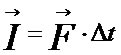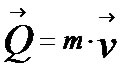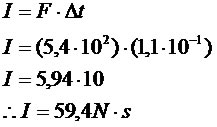Mechanics

# Impulse and Momentum

Brazilian national team player

When a soccer player kicks a ball, he applies a force for a certain amount of time on the ball. This action is directly related to the distance that the object will travel in a given time.

Impulse of a force

Let a constant force F act on a body during a time interval ?t. The impulse I of this constant force in this time interval is a vector quantity defined by:The unit of measure of impulse in the international system (SI) is Newton x seconds (Ns) Momentum

Consider

a body of mass m, moving with a velocity v, with respect to a reference frame. We say that the momentum of the body is the product of its mass and velocity:Where:
Q is the momentum (vector quantity)
m is the mass of the body (given in kg in SI)
v is the velocity (given vector quantity in m/s in SI)

In the international system, momentum is given in kg.m/s (kilogram x meter per second).

Examples:

1) A force of magnitude 5.4 x 102 N is applied to a material point, during a time interval equal to 1.1 x 10-1s. Determine the magnitude of the impulse of the force applied at the material point.2) Consider a small sphere of mass 2.0 kg, which at a given instant has a horizontal velocity, oriented from left to right and of magnitude 5.0 m/s. Determine the magnitude, direction, and direction of momentum of this sphere.

The direction of the momentum vector is the same as the velocity. (horizontal)
The direction of momentum vector is from left to right.